# Comp2FlowExchangeReaction

Model number
0248

Model with two species A and B, with flow in a plasma compartment and exchange with an interstitial fluid compartment with A converting to B reversibly.

## Description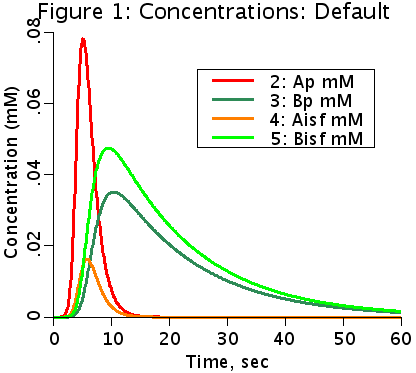This is a two compartment model (plasma and isf) exchange model with flow in the plasma compartment. Both spaces are instantaneously well mixed. A and B reversibly convert to each other in the isf space. The isf space can also be used as a cell space. Flow, Fp, and exchange rates, PSa and PSb, have the same units, ml/(g*min) (milliliters per minute per gram of tissue). These units are used in the physiological terminology to relate them to fluxes per gram of tissue.
The conversion rates have the same units, ml/(g*min). Ga2b is the conversion rate of A going to B, and Gb2a is the conversion rate of B becoming A.
The steady state solutions for constant inflow of A are solved implicitly, using the final value of the input concentration (assumed to have been constant).

## Equations

#### Ordinary Differential Equations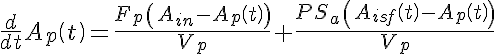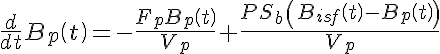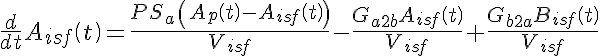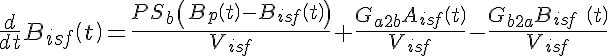#### Initial Conditions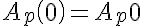,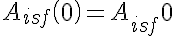,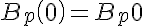, and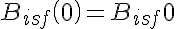.

The equations for this model may be viewed by running the JSim model applet and clicking on the Source tab at the bottom left of JSim's Run Time graphical user interface. The equations are written in JSim's Mathematical Modeling Language (MML). See the Introduction to MML and the MML Reference Manual. Additional documentation for MML can be found by using the search option at the Physiome home page.

• Download JSim model MML code (text):
• Download translated SBML version of model (if available):
References

None.

Key terms
Course
compartment
compartmental
tutorial
exchange
multiple compartments
flux